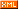# zyl910

:: :: :: :: :: :::: ::192 随笔 :: 0 文章 :: 115 评论 :: 0 引用

File:      StudyRGB2Gray.txt
Name:      彩色转灰度算法彻底学习
Author:    zyl910
Version:   V1.0
Updata:    2006-5-22

对于彩色转灰度，有一个很著名的心理学公式：
Gray = R*0.299 + G*0.587 + B*0.114

而实际应用时，希望避免低速的浮点运算，所以需要整数算法。
注意到系数都是3位精度的没有，我们可以将它们缩放1000倍来实现整数运算算法：
Gray = (R*299 + G*587 + B*114 + 500) / 1000

RGB一般是8位精度，现在缩放1000倍，所以上面的运算是32位整型的运算。注意后面那个除法是整数除法，所以需要加上500来实现四舍五入。
就是由于该算法需要32位运算，所以该公式的另一个变种很流行：
Gray = (R*30 + G*59 + B*11 + 50) / 100

但是，虽说上一个公式是32位整数运算，但是根据80x86体系的整数乘除指令的特点，是可以用16位整数乘除指令来运算的。而且现在32位早普及了（AMD64都出来了），所以推荐使用上一个公式。

上面的整数算法已经很快了，但是有一点仍制约速度，就是最后的那个除法。移位比除法快多了，所以可以将系数缩放成 2的整数幂。
习惯上使用16位精度，2的16次幂是65536，所以这样计算系数：
0.299 * 65536 = 19595.264 ≈ 19595
0.587 * 65536 + (0.264) = 38469.632 + 0.264 = 38469.896 ≈ 38469
0.114 * 65536 + (0.896) =  7471.104 + 0.896 = 7472

可能很多人看见了，我所使用的舍入方式不是四舍五入。四舍五入会有较大的误差，应该将以前的计算结果的误差一起计算进去，舍入方式是去尾法：

写成表达式是：
Gray = (R*19595 + G*38469 + B*7472) >> 16

2至20位精度的系数：
Gray = (R*1 + G*2 + B*1) >> 2
Gray = (R*2 + G*5 + B*1) >> 3
Gray = (R*4 + G*10 + B*2) >> 4
Gray = (R*9 + G*19 + B*4) >> 5
Gray = (R*19 + G*37 + B*8) >> 6
Gray = (R*38 + G*75 + B*15) >> 7
Gray = (R*76 + G*150 + B*30) >> 8
Gray = (R*153 + G*300 + B*59) >> 9
Gray = (R*306 + G*601 + B*117) >> 10
Gray = (R*612 + G*1202 + B*234) >> 11
Gray = (R*1224 + G*2405 + B*467) >> 12
Gray = (R*2449 + G*4809 + B*934) >> 13
Gray = (R*4898 + G*9618 + B*1868) >> 14
Gray = (R*9797 + G*19235 + B*3736) >> 15
Gray = (R*19595 + G*38469 + B*7472) >> 16
Gray = (R*39190 + G*76939 + B*14943) >> 17
Gray = (R*78381 + G*153878 + B*29885) >> 18
Gray = (R*156762 + G*307757 + B*59769) >> 19
Gray = (R*313524 + G*615514 + B*119538) >> 20

仔细观察上面的表格，这些精度实际上是一样的：3与4、7与8、10与11、13与14、19与20
所以16位运算下最好的计算公式是使用7位精度，比先前那个系数缩放100倍的精度高，而且速度快：
Gray = (R*38 + G*75 + B*15) >> 7

其实最有意思的还是那个2位精度的，完全可以移位优化：
Gray = (R + (WORD)G<<1 + B) >> 2

由于误差很大，所以做图像处理绝不用该公式（最常用的是16位精度）。但对于游戏编程，场景经常变化，用户一般不可能观察到颜色的细微差别，所以最常用的是2位精度。

posted on 2006-05-22 17:56 zyl910 阅读(...) 评论(...) 编辑 收藏